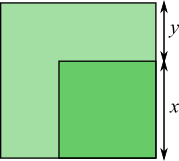#### You may also like### Golden Thoughts

Rectangle PQRS has X and Y on the edges. Triangles PQY, YRX and XSP have equal areas. Prove X and Y divide the sides of PQRS in the golden ratio.### Two Cubes

Two cubes, each with integral side lengths, have a combined volume equal to the total of the lengths of their edges. How big are the cubes? [If you find a result by 'trial and error' you'll need to prove you have found all possible solutions.]### Square Mean

Is the mean of the squares of two numbers greater than, or less than, the square of their means?

# A Third of the Area

##### Age 14 to 16 ShortChallenge Level

In this diagram, the area of the small square is $\dfrac13$ of the area of the large square.

Find $\dfrac xy$.This problem is taken from the World Mathematics Championships
You can find more short problems, arranged by curriculum topic, in our short problems collection.